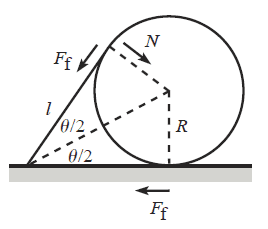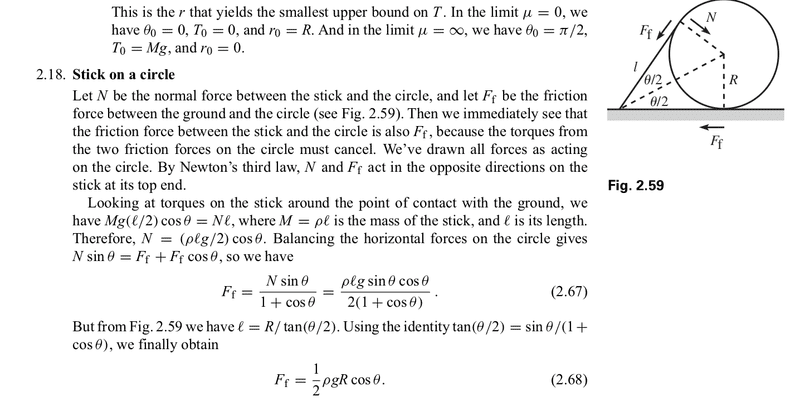# Coefficient of friction between stick and circle

Demetrius

## Homework Statement

Using the results from problem 2.18 for the setup shown in the Figure below show that if the system is to remain at rest, then the coefficient of friction:

a) between the stick and the ground must satisfy

$$μ ≥ \frac {sin(Θ)cos(Θ)} {(1+cos(Θ))(2-cos(Θ))}$$## Homework Equations

The result from 2.18 is:

$$F_f = \frac 1 2 ρgRcos(Θ)$$

Which is the friction between the stick and the circle.

## The Attempt at a Solution

The sum of torques on the stick is (my origin is the point where the stick and circle is touching):

## \frac L 2 mgcos(Θ) + L F_fsin(Θ) = L Ncos(Θ) ##

This is how far I am.

haruspex
Homework Helper
Gold Member
2020 Award
I am puzzled by the use of "Ff". The diagram uses that for two different forces, and your equation uses it for a third. Which of these is intended in the 2.18 result is unclear.
What is ρ?
What is known about the masses of the stick and the cylinder/ball?

Demetrius
ρ is the mass density of the stick. ##F_f## is the friction force. The complete solution of 2.18 is below:haruspex
Homework Helper
Gold Member
2020 Award
ρ is the mass density of the stick. ##F_f## is the friction force. The complete solution of 2.18 is below:

View attachment 210750
That helps, and I can see that the frictional force from ground on stick must also be Ff.
What about N? Is there some simple argument that the two normal forces on the stick are the same?
What other equations do you have for forces on the stick?

Demetrius
Honestly before your response, I thought the two normal forces was different but if I had to come up with an argument of why they are the same then here it is:

Okay there is a normal force from the ground on the stick and there is a normal force from the circle on the stick. The only argument I can provide that these two normal forces are the same is that Normal force is a perpendicular force a surface applies to an object. The magnitude of the force is dependent on the object not the surface. So even though the two normal forces are from two different surfaces, the magnitude must be same. That is the only argument I can provide?

haruspex
Homework Helper
Gold Member
2020 Award
Honestly before your response, I thought the two normal forces was different but if I had to come up with an argument of why they are the same then here it is:

Okay there is a normal force from the ground on the stick and there is a normal force from the circle on the stick. The only argument I can provide that these two normal forces are the same is that Normal force is a perpendicular force a surface applies to an object. The magnitude of the force is dependent on the object not the surface. So even though the two normal forces are from two different surfaces, the magnitude must be same. That is the only argument I can provide?
No, I did not mean to imply they are the same, they are not. I am querying your use of the same name for two different normal forces.

Demetrius
No, I did not mean to imply they are the same, they are not. I am querying your use of the same name for two different normal forces.

That is a mistake, the normal force I am referring to is the normal force from the ground on the stick. Here is my revised torque equation:

$$\frac L 2 mgcos(Θ) + L F_fsin(Θ) = L N_gcos(Θ)$$

where ##N_g## is the normal force from the ground on the stick

haruspex
Homework Helper
Gold Member
2020 Award
That is a mistake, the normal force I am referring to is the normal force from the ground on the stick. Here is my revised torque equation:

$$\frac L 2 mgcos(Θ) + L F_fsin(Θ) = L N_gcos(Θ)$$

where ##N_g## is the normal force from the ground on the stick
That's better.

Demetrius
Okay, well this is the part I am having trouble with, but here are the sum of forces on the stick in the horizontal tradition. I have chosen a traditional axis where the positive x is pointing from the point of contact between the stick and ground and toward the circle:

$$Σ F_x = F_f + N_gsin(\theta) - N_csin(\theta) - F_{f,c}cos(\theta) - F_gsin(\theta)$$

where ##N_c## is the normal force between the circle and ground. ##F_{f,c}## is the friction force between the circle and the stick

Demetrius
I can see that the frictional force from ground on stick must also be Ff

Question, you said earlier that the friction force between the stick and the ground is the same as the friction force between the stick and the circle. I said the same thing to my classmate and he disagreed. My reasoning for believing these friction forces must be the same was:

to keep the system static. The torque caused by the friction forces must be same because if they were not then the stick will move.

I could not proved this with math and I was not 100% sure if this was right either. So, are the two friction forces equal and how so?

haruspex
Homework Helper
Gold Member
2020 Award
are the two friction forces equal and how so?
Consider horizontal forces on the stick+circle system.

Demetrius
Okay I understand now. The only horizontal forces on the stick+circle system is the friction forces...... Is my horizontal forces on the stick correct:

$$Σ F_x = F_f + N_gsin(\theta) - N_csin(\theta) - F_{f,c}cos(\theta) - F_gsin(\theta)$$

where ##N_c## is the normal force between the circle and ground. ##F_{f,c}## is the friction force between the circle and the stick

haruspex
Homework Helper
Gold Member
2020 Award
Okay I understand now. The only horizontal forces on the stick+circle system is the friction forces...... Is my horizontal forces on the stick correct:

$$Σ F_x = F_f + N_gsin(\theta) - N_csin(\theta) - F_{f,c}cos(\theta) - F_gsin(\theta)$$

where ##N_c## is the normal force between the circle and ground. ##F_{f,c}## is the friction force between the circle and the stick
How does the normal force from the ground have a horizontal component?
Since we have established all three frictional forces are equal, no need to distinguish them with different subscripts.

Demetrius
Well the normal force from the ground is perpendicular to the ground (or surface). Hence it is not perpendicular to the stick right?

haruspex
Homework Helper
Gold Member
2020 Award
Well the normal force from the ground is perpendicular to the ground (or surface). Hence it is not perpendicular to the stick right?
Right, but your equation was supposed to be for horizontal forces on the stick, not forces along the stick.

Demetrius
Right, but your equation was supposed to be for horizontal forces on the stick, not forces along the stick.

Okay, now I understand. The normal force from the ground does not have a horizontal component because the force is acting on the stick in only one direction and that is the vertical direction.

Here is my revised equation for horizontal forces on the stick:

$$Σ F_x = F_f - N_csin(\theta) - F_fcos(\theta)$$

Last edited by a moderator:
haruspex
$$Σ F_x = F_f - N_csin(\theta) - F_fcos(\theta)$$